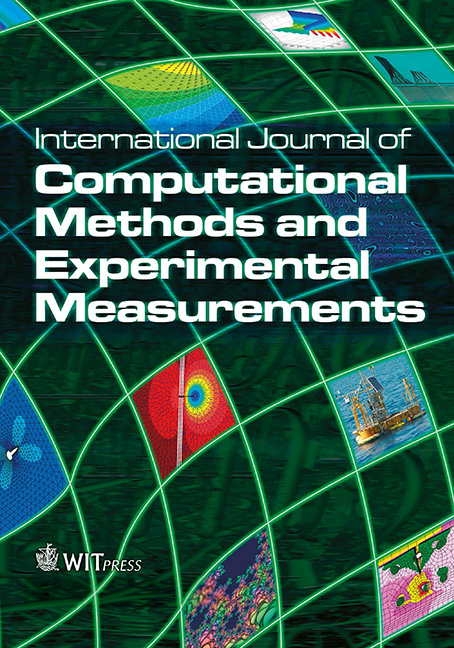WIT Press

Modeling mixed boundary problems with the complex variable boundary element method (CVBEM) using Matlab and Mathematica

Price

Free (open access)Volume

Volume 3 (2015), Issue 3

Pages

9

Page Range

269 - 278

Paper DOI

10.2495/CMEM-V3-N3-269-278

WIT Press

Author(s)

ANTHONY N. JOHNSON, T.V. HROMADKA II, M.T. HUGHES & S.B. HORTON

Abstract

The complex variable boundary element method or CVBEM is a numerical technique that can provide solutions to potential value problems in two or more dimensions by the use of an approximation function that is derived from the Cauchy integral equation in complex analysis. Given the potential values (i.e. a Dirichlet problem) along the boundary, the typical problem is to use the potential function to solve the governing Laplace equation. In this approach, it is not necessary to know the streamline values on the boundary. The modeling approach can be extended to problems where the streamline function is needed because there are known streamline values along the problem boundary (i.e. a mixed boundary value problem). Two common problems that have such conditions are insulation on a boundary and fluid flow around a solid obstacle. In this paper, five advances in the CVBEM are made with respect to the modeling of the mixed boundary value problem; namely (1) the use of Mathematica and Matlab  in tandem to calculate and plot the flow net of a boundary value problem. (2) The magnitude of the size of the problem domain is extended. (3) The modeling results include direct computation and development of a flow net. (4) The graphical displays of the total flownet are developed simultaneously. And (5) the nodal point location as an additional degree of freedom in the CVBEM modeling approach is extended to mixed boundaries. A demonstration problem of fluid flow is included to illustrate the flownet development capability.

Keywords

approximate boundary, collocation, complex variable boundary element method (CVBEM), complex variables, mixed boundary conditions, Mathematica, Matlab, MATLink